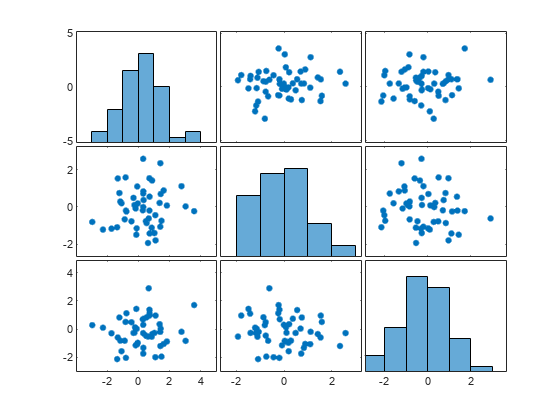November 10 2022

## Agenda

• What is multidimensional data?
• Strategies for Multidimensional data visualization:
• direct visualization
• projections

## Multidimensional data

Real data is often high-dimensional (i.e. contain more than 3 features) – visualizations can help understand this type of data.

“For human perception, the data must be represented in a low-dimensional space, usually of two or three dimensions”

(Dzemyda, Kurasova, & Zilinskas, 2012)

## Direct Visualization

“The direct visualization methods do not have any defined formal mathematical criterion for estimating the visualization quality”

(Dzemyda, Kurasova, & Zilinskas, 2012)

Example: Scatter plot matrix

## Scatter plot matrix

• A grid (or matrix) of scatterplots showing bivariate relationships – histograms often accompany the scatterplots showing the distribution of values for individual variables.## Histogram Building

We will first create a histogram, since we already built many scatterplots before. For that, we need to use the following transforms:

• Extent Transform: to calculate the min and max values of a variable
• Bin Transform: to calculate bins to place each observation

We then count how many observations for bins.

## Extent Transform

We will read the data in and use an extent transform to calculate the min and max values of the calories variable. We will save the results of the transform to a signal called calories_max_min

data: [
{
format: { type: "csv"},
transform: [
{
type: "extent",
field: "calories",
signal: "calories_max_min"
}
]
}
]

## Extend Transform

You can accessed the values from the JavaScript console in your browser.

view.signal("calories_max_min");

## Bin Transform

We then use a bin transform with the signal we created to calculate bins.

{
type: "bin",
signal: "bins",
field: "calories",
extent: { signal: "calories_max_min" },
maxbins: 20

}

Two variables are added to the data: bin0 and bin1 – use view.data("menu"); on the JavaScript console to inspect the data.

## Bins

Let’s now create a new data set where we can how many observations per bin.

{
name: "calories_bins",
transform: [
{
type: "aggregate",
groupby: ["bin0", "bin1"]
}
]
}

Inspect the new data set using view.data("calories_bins");

## Scales

scales: [
{
name: "xScale",
type: "linear",
bins: { signal: "bins" },
domain: { signal: "[bins.start, bins.stop]" },
range: "width"

},
{
name: "yScale",
type: "linear",
domain: { data: "calories_bins", field: "count" },
range: "height"
}
],

## Marks

marks: [
{
type: "rect",
from: { data: "calories_bins" },
encode: {
enter: {
x: { field: "bin0", scale: "xScale" },
x2: { field: "bin1", scale: "xScale" },
y: { field: "count", scale: "yScale" },
y2: { value: 0, scale: "yScale" }
}
}
}
]